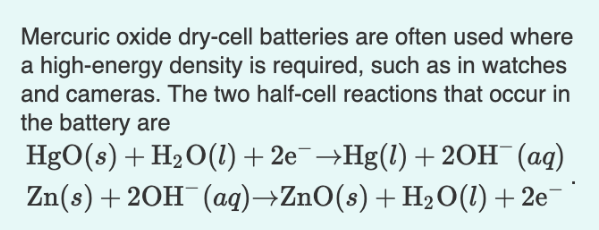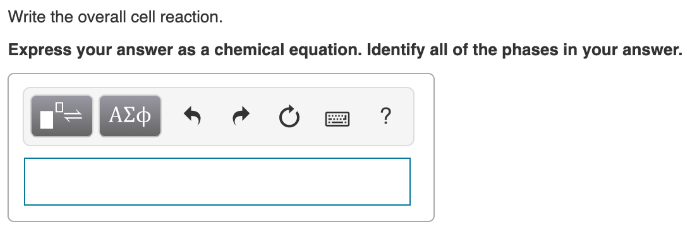# Mercuric oxide dry-cell batteries are often used where a high-energy density is required, such as in watches and cameras. The two half-cell reactions that occur in the battery are HgO(s) + H2O(l) + 2e^- → Hg(l) + 2OH^-(aq) Zn(s) + 2OH^-(aq) → ZnO(s) + H2O(l) + 2e^-. Write the overall cell reaction. Express your answer as a chemical equation. Identify all of the phases in your answer.